Related Articles

# Differentiability of a Function | Class 12 Maths

• Difficulty Level : Expert
• Last Updated : 04 Dec, 2020

Continuity or continuous which means, “a function is continuous at its domain if its graph is a curve without breaks or jumps”. A function is continuous at a point in its domain if its graph does not have breaks or jumps in the immediate neighborhood of the point.

Continuity at a Point:

A function f(x) is said to be continuous at a point x = a of its domain, if

(LHL) = (RHL) = f(a) or lim f(x) = f(a)

where

(LHL)x = a  = limx -> a f(x) and

(RHL)x = a = limx -> a+ f(x)

Note: To evaluate LHL and RHL of a function f(x) at x = a, put x – h and x + h respectively, where h -> 0

The discontinuity at a Point:

If f(x) is not continuous at a point x = a, then it is discontinuous at x = a.

There are various types of discontinuity:

• Removable discontinuity: If limx -> a f(x) = limx -> a+ f(x) ≠ f(a)
• Discontinuity of the first kind: If limx -> a+ f(x) ≠ limx -> a+ f(x)
• Discontinuity of the second kind: If limx -> a f(x) or limx -> a+ f(x) both do not exist

### Differentiability and Concept of Differentiability

A function f(x) is said to be differentiable at a point x = a, If Left hand derivative at (x = a) equals to Right hand derivative at (x = a) i.e

LHD at(x = a) = RHD at (x = a),

where

Right hand derivative, Rf’ (a) = limh->0 (f(a + h) – f(a)) / h and

Left hand derivative Lf’ (a) = limh->0 (f(a – h) – f(a)) / -h

Note: The common value of Rf’ (a) and Lf’ (a)  is denoted by f'(a) and it is known as the derivative of f(x) at x = a. Every differentiable function is continuous but every continuous function need not be differentiable.

### Conditions of Differentiability

Condition 1: The function should be continuous at the point. As shown in the below image.

Have like this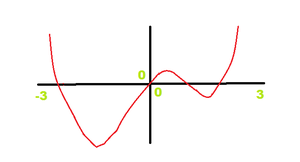Don’t have this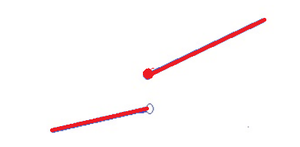Condition 2: The graph does not have a sharp corner at the point as shown below.

Doesn’t have a sharp corner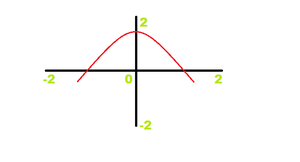Having the sharp curve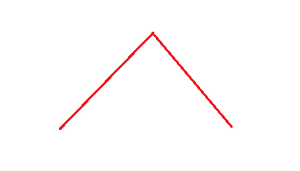Condition 3: The graph does not have a vertical line at the point. The graph doesn’t have the vertical line as shown in the below figure indicated by the circle.

Indicating the vertical line in the graph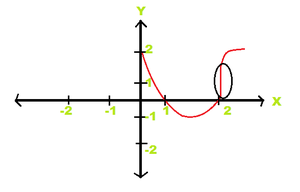Note: The relationship between continuity and differentiability is that all differentiable functions happen to be continuous but not all continuous functions can be said to be differentiable. Let’s discuss Differentiability and Continuity.

### Differentiability of Special Functions

Lets consider some special functions are:

1. f(x) = [x], which is the greatest integer of x, and the other one
2. f(x) = {x}, which is the fractional part of x

1. For f(x) = [x]

So, first, we go with f(x) = [x], to check the differentiability of the function we have to plot the graph first. So let’s plot the graph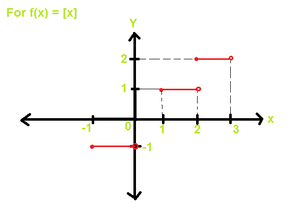So as we see in the graph that between 0 and 1 the value of the function is 0 and between 1 and 2 the value of the function is 1 and between 2 and 3 the value of the function is 2, Similarly on the -ve side between -1 and 0 the value of the function is -1. So if we talk about the domain of the function the domain is the entire value of real values but the range of this function is only integers, the function will take only integer values because it is the greatest integer of x. Now let us talk about the differentiability of this particular function. Firstly we are talking about integer points, so firstly we are checking the differentiability at integer points. As we know for the function to be differentiable the function should continuous first, as we see the graph at point x = 1,

At integer point,

Consider x =1

RHL = limx -> 1+ [x] = 1

LHL = limx -> 1 [x] = 0

So, RHL ≠ LHL

It is checked for x = 1, but it will valid for all the integer points result will be the same. [x] is not continuous at integer points, So it is not differentiable at integer points. So, [x] is not continuous at integer points. It is also not differentiable at integer points.

Now, let us find what is happening on non-integer points. Let us consider x = 2.5, let’s find RHL and LHL

RHL = limx -> 2.5+ [x] = 2

LHL = limx -> 2.5 [x] = 2

Since both RHL and LHL are equal, so our function [x] is continuous at a non-integer point. Now we have to check the differentiability at non-integer points, so we have to find the slope of the function which we can find by finding the derivative of the function [x] at point 2.5

f'(x) = d[x] / dx at x = 2.5 = 0

Therefore, the function is differentiable at all non-integer points.

2. For f(x) = {x}

Now we are considering the second function which f(x) = {x} which is the fractional part of x. To find the differentiability and continuity we have to plot the graph first.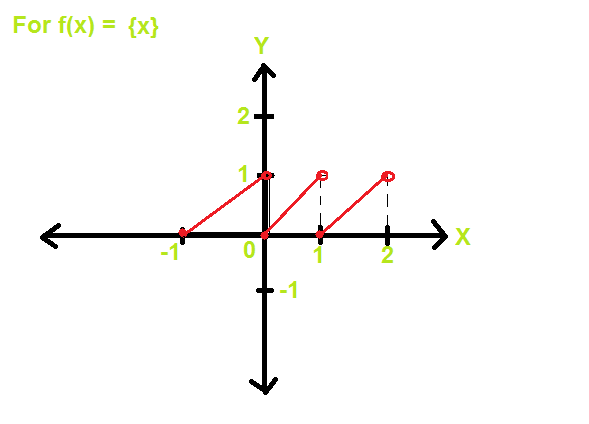So in this graph, the domain of the function is the entire range of real values and the range of this function is only 0 to 1 because any fractional part of the value is between 0 to 1. Let’s find for integer values, Consider the point x = 1

RHL = limx -> 1+ {x} = 0

LHL = limx -> 1 {x} = 1

Since RHL ≠ LHL function {x} is not continuous and hence not differentiable. Let us find for non-integer points. Considering x = 1.5

RHL = limx -> 1.5+ {x} = 0.5

LHL = limx -> 1.5 {x} = 0.5

Since RHL = LHL, function is continuous. To find the differentiability we have to find the slope of the function which we can find by finding the derivative of the function [x] at point 2.5

f'(x) = d{x} / dx at x = 1.5 = 1

Therefore, the function {x} is differentiable at non-integer points.

### Problems On Differentiability

Problem 1: Prove that the greatest integer function defined by f(x) = [x] , 0 < x < 3 is not differentiable at x = 1 and x = 2.

Solution:

As the question given  f(x) = [x] where x is greater than 0 and also less than 3. So we have to check the function is differentiable at point x =1 and at x = 2 or not. To check the differentiability of function, as we discussed above in Differentiation that LHD at(x=a) = RHD at (x=a) which means,

Lf’ at (x = a) = Rf’ at (x = a) if they are not equal after solving and putting the value of a in place of x then our function should not differentiable and if they both comes equal then we can say that the function is differentiable at x = a, we have to solve for two points x = 1 and x = 2. Now, let’s solve for x = 1

f(x) = [x]

Put x = 1 + h

Rf’ = limh -> 0 f(1 + h) – f(1)

= limh -> 0 [1 + h] – 

Since [h + 1] = 1

= limh -> 0 (1 – 1) / h = 0

Lf'(1) = limh -> 0 [f(1 – h) – f(1)] / -h

= limh -> 0 ( [1 – h] –  ) / -h

Since [1 – h] = 0

= limh -> 0 (0 – 1) / -h

= -1 / -0

= ∞

From the above solution it is seen that Rf’ ≠ Lf’, so function f(x) = [x] is not differentiable at x = 1. Now, let’s check for x = 2. As we solved for x = 1 in the same we are going to solve for x = 2. The condition should be the same we have to check that, Lf’ at (x = 2) = Rf’ at (x = 2) or not if they are equal then our function is differentiable at x = 2 and if they are not equal our function is not differentiable at x = 2. So, let’s solve.

f(x) = [x]

Differentiability at x=2

Put x = 2+h

Rf'(1) = limh -> 0 f(2 + h) – f(2)

= limh -> 0 ([2 + h] – ) / h

Since 2 + h = 2

= limh -> 0 (2 – 2) / h

= limh -> 0 0 / h

=0

Lf'(1) = limh -> 0 (f(2 – h) – f(2)) / -h

= limh -> 0 ([2 – h] – ) / -h

= limh -> 0 (1 – 2) / -h

Since [2 – h] =1

= -1 / -0

= ∞

From the above solution it is seen that Rf'(2) ≠ Lf'(2), so f(x) = [x] is not differentiable at x = 

Problem 2:Show that the above function is not derivable at x = 0.

Solution:

As we know to check the differentiability we have to find out Lf’ and Rf’ then after comparing them we get to know that the function is differentiable at the given point or not. So let’s first find the Rf'(0).

Rf'(0) = limh -> 0 f(0 + h) – f(0)

= limh -> 0 (f(h) – f(0)) / h

= limh -> 0 h . [{(e(1 / h) – 1) / (e(1 / h) + 1) } – 0]/h

= limh -> 0 (e(1 / h) – 1) / (e(1 / h) + 1)

Multiply by e(-1 / h)

= limh -> 0 {1 – e(-1 / h) / 1 + e(-1 / h)

= (1 – 0) / (1 + 0)

= 1

After solving we had find the value of Rf'(0) is 1. Now after this let’s find out the Lf'(0) and then we will check that the function is differentiable or not.

Lf'(0) = limh -> 0 { f(0 – h) – f(0) } / -h

= limh -> 0 -h . [{e(-1 / h) – 1 / e(-1 / h) + 1} – 0] / -h

= limh -> 0 { (e(-1 / h) – 1) / (e(-1 / h) + 1) }

= limh -> 0 { (1 – e(-∞)) / (1+e(-∞))}

= (0 – 1) / (0 + 1)

= -1

As we saw after solving Lf'(0) the value we get -1. Now checking if the function is differentiable or not, Rf'(0) ≠ Lf'(0) (-1≠1). Since Rf'(0) ≠ Lf'(0), so f(x) is not differentiable at x = 0.

Problem 3: A function is f(x) defined by

f(x) = 1 + x of x < 2

f(x) = 5 – x of x ≥ 2

If function f(x) differentiable at x = 2?

Solution:

So, for finding Lf'(2) we take the function f(x) = 1 = x, in the same way for finding Rf'(2) we take the function f(x) = (5 – x). Let’s find out Lf'(2) and Rf'(2)

Lf'(2) = limh -> 0 {f(2 – h) – f(2)} / -h

= limh -> 0 [[(2 – h) + 1] – [5 – 2]] / -h

= limh -> 0 (3 – h – 3) / -h

= limh -> 0 -h / h

= -1

Rf'(2) = limh -> 0 {f(2 + h) – f(2)} / h

= limh -> 0 [[5 – (2 + h)] – 3] / h

=limh -> 0 h / h

= 1

In the first line Lf'(2) after putting in the formula, for f(2) we are putting second function (5 – x). After solving the Lf'(2) we get the value -1. For calculating Rf'(2) we are using the second function 5-x and putting in the formula of Rf’, on solving the Rf'(2) we get the value 1. Since, Rf'(2) ≠ Lf'(2) so we can say the function f(x) is not differentiable at x = 2.

Problem 4: Find whether the following function is differentiable at x = 1 and x = 2 or not?

f(x) = x , x < 1

f(x) = 2 – x , 1 ≤ x ≤ 2

f(x) = -2 + 3x – x2 , x > 2

Solution:

So, to check the differentiability at x = 1. We have to find out the Lf'(1) and Rf'(1). So let’s find Lf'(1) and Rf'(1). For solving we are putting the value of x which is 1 in the formula of Lf’ and Rf’. For solving Lf’ we are taking 1st function which is x because we are calculating for left-hand derivative. After putting simply solve for Lf'(1) we get the value 1. For solving Rf’ we are taking the 2cd function which is 2 – x because we are calculating for right hand derivative. After putting, simply solve for Rf'(1) we get the value -1. So, Rf'(1) ≠ Lf'(1). Hence function is not differentiable at x= 1. Now, let’s check for x = 2

Lf'(2) = limh -> 0 {f(2 – h) – f(2)} / -h

= limh -> 0 {2 – (2 – h) – (2 – 2)} / -h

= limh -> 0 h / -h

= -1

Rf'(2) = limh -> 0 { f(2 + h) – f(2)

= limh -> 0 {-2 + 3(2 + h) – (2 + h)2 – (2 – 2) } / h

= limh -> 0 {-2 + 6 + 3h – (4 + h2 + 4h) – 0} / h

= limh -> 0 (-h2 – h) / h

= limh -> 0 -h . (h + 1) / h

= – (0 + 1)

= -1

For solving Lf'(2) we are taking the (2 – x) function because this function is less than 2, and as we know for finding the left-hand derivative we have to take the function which is les than the given limit. So, after putting in the formula of Lf’, solving the problem, and after solving we get the value -1. Now, for solving Rf’, we are taking -2 + 3x – x2, as we know for finding Right-hand derivative the limit should be greater than the point at which we are calculating. Now put in the formula o Rf’ and we get the value after solving is -1. So, as we saw that the Rf'(2) = Lf'(2). Hence the function f(x) is differentiable at x = 2.

Attention reader! Don’t stop learning now. Join the First-Step-to-DSA Course for Class 9 to 12 students , specifically designed to introduce data structures and algorithms to the class 9 to 12 students

My Personal Notes arrow_drop_up